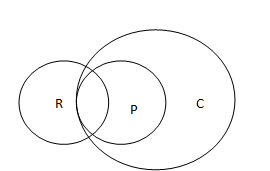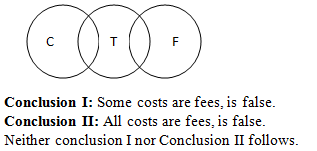# Paytm Reasoning Question

DIRECTIONS for questions 1-3: What should come in place of question mark (?) in the following number/alphabetic series.
1. 12  34  54  71  84  ?
1. 89
2. 92
3. 124
4. 102
5. 110
The respective differences between the terms : 22, 20, 17, 13
The difference between differences : 2, 3, 4 & 5
=>The difference is 18 & the required no. = 84 + 8 = 92.
2. 360  72  18  6  ?  3
1. 3
2. 5
3. 4
4. 4.5
5. 6
The pattern is as follows:
360/5 = 72
72/4 = 18
18/3 = 6
=>6/2 = 3.
1. 382  380  374  356  302 ?
1. 212
2. 240
3. 140
4. 201
5. 158
The pattern is as follows:
382 – 2 = 380
380 – 6 = 374
374 – 18 = 356
356 – 54 = 302
=>302 – 162 = 140.
DIRECTIONS for questions 4 to 6: The questions below has two/three statements followed by two conclusions numbered I and II. You have to take the given statements to be true even if they seem to be at variance with commonly known facts. Read all the conclusions and then decide which of the given conclusions logically follow from the given statements disregarding commonly known facts.
1. If only (1) conclusion follows
2. If only (2) conclusion follows
3. If either (1) or (2) follows
4. If neither (1) nor (2) follows
5. If both (1) and (2) follow
2. Statements: All features are attributes. All attributes are traits.
Conclusion I: All traits are attributes.
Conclusion II: All features are traits3. Statements: Some rates are prices. All prices are charges.
Conclusion I: Atleast some rates are charges.
Conclusion II: All charges are pricesConclusion I:Atleast some rates are charges, is true.
Conclusion II: All charges are prices, is false.
Only I conclusion follows.
4. Statements: Some costs are tariffs. Some tariffs are fees
Conclusion I: Some costs are fees.
Conclusion II: All costs are fees.5. What should come in place of the question mark (?) in the following series based on the given arrangement?
1. EZ
2. LV
3. EL
4. @L
5.  6L
The pairs are made as: the first and the sixth, the second pair will be the preceding symbol to the sixth and the one which is fifth to it, the third pair will be the preceding symbol to the fifth and the one which is fourth to it, the fourth pair will be the preceding symbol to the fifth and the one which is third to it. Similarly, Next pair will be preceding symbol of Z which is @ and the one which is second to it. Therefore, @L will be next pair.
6. The following series is based on a particular combination of English alphabets and numbers. Which of the following will come in place of question mark (?) in the given series?
9Z-20 11A-18 13Y-16 15B-14 ?
1. 14D-10
2. 16B-12
3. 17X-12
4. 16X-10
5. 17D-10
9Z-20   11A-18    13Y-16    15B-14     ?
In this , first number of each Pair is increasing by 2 and the last number is decreasing by 2such as
9_ -20  ,   11_-18 ,   13_-16    15_-14   , so that next arrangement will be
15+2_- 14-2=> 17_-12
two series are appear as alternatively with alphabetical series and reverse alphabetical series, Such as
Z    , A    , Y     , B    Therefore, next letter will be X. Combining both numeric and alphabetical series the next pair will be = 17X-12
7. How many such pairs of digits are there in the number 31748296 (both in forward and backward directions), each of which has as many digits between them as in the arithmetic series?
1. Three
2.  More than three
3.  One
4.  None
5. Two